# Subject : Mathematics

## Topic : POLYGONS AND PLANE FIGURES

Behavioural Objectives :  At the end of the lesson, the pupils should be able to

• Explain the meaning of polygons
• Give examples of polygons
• Mention types of polygons
• Say the properties of polygons

Previous Knowledge : Pupils have previous knowledge of    TYPES OF ANGLES   that was taught in the previous lesson

Instructional Materials :

• Pictures
• Wall Posters
• Related Online Videos
• Role Playing

Reference Materials

• Scheme of Work
• Online Information
• Textbooks
• Workbooks
• 9 Year Basic Education Curriculum

Content :

POLYGON AND PLANE FIGURES

A polygon is a shape with straight sides. Polygons are shapes . Shapes are the appearance or look of things There are regular polygons and irregular polygons . Squares , rectangles , Triangles and quadrilaterals are polygons. Some

other polygons have special names.

Number of straight lines or sides in polygons

3 Triangle

4 Quadrilateral

5 Pentagon

6 Hexagon

7 Heptagon

8 Octagon

Regular polygon

A polygon is said to be regular if all of its sides are equal.

Also all its interior angles are

equal.

Consequently all exterior angles are also equal.

Irregular polygon

If all the interior angles are not equal, then the polygon is not regular. It is then known as an

irregular polygon.

Study the table drawn below:

Name of Polygon No of sides No of angles. No of triangles

Triangle. 3 3 1

Quadrilateral. 4. 4. 2

Pentagon 5. 5. 3

Hexagon. 6. 6 4

Heptagon. 7 7 5

Octagon. 8. 8. 6

Triangles

Triangles can be classified by sides or angles.

Draw three triangles on your not book. Name them as ∆PQR, ∆ABC and ∆LMN. With the help of protector measure all the angles the angles and find them:

In ∆ABC

∠ABC + ∠BCA + ∠CAB = 180°.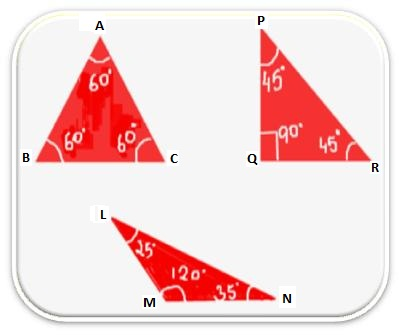In ∆PQR

∠PQR + ∠QRP + ∠RPQ = 180°

In ∆LMN

∠LMN + ∠MNL + ∠NLM = 180°

Angle Properties of Triangles

Here, we observe that in each case, the sum of the measures of three angles of a triangle is 180°.

Hence, the sum of the three angles of a triangle is equals to 180°.

For Example:

1. In a right triangle, if one angle is 50°, find its third angle.

Solution:

∆ PQR is a right triangle, that is, one angle is right angle.

Given, ∠PQR = 90°

∠QPR = 50°

Therefore, ∠QRP = 180° – (∠Q + ∠ P)

= 180° – (90° + 50°)

= 180° – 140°

∠R = 40°

2. Draw a ∆ABC. Measure the length of its three sides. Let the lengths of the three sides be AB = 5 cm, BC = 7 cm, AC = 8 cm. Now add the lengths of any two sides compare this sum with the lengths of the third side.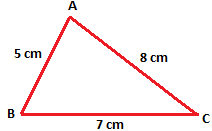(i) AB + BC = 5 cm + 7 cm = 12 cm

Since 12 cm > 8 cm

Therefore, (AB + BC) > AC

(ii) BC + CA = 7 cm + 8 cm = 15 cm

Since 15 cm > 5 cm

Therefore, (BC + CA) > AB

(iii) CA + AB = 8 cm + 5 cm = 13 cm

Since 13 cm > 7 cm

Therefore, (CA + AB) > BC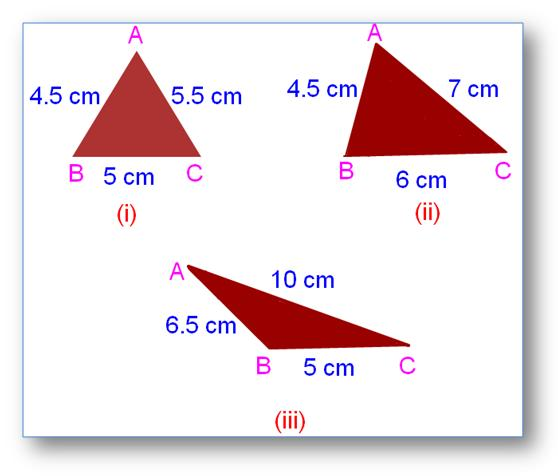two sides of a triangle is greater than the length of the third side.

For Example:

1. Is it possible to have a triangle whose sides are 5 cm, 6 cm and 4 cm?

Solution:

The lengths of the sides are 5 cm, 6 cm, 4 cm,

(a) 5 cm + 6 cm > 4 cm.

(b) 6 cm + 4 cm > 5 cm.

(c) 5 cm + 4 cm > 6cm.

Hence, a triangle with these sides is possible.

We will solve some of the examples of properties of triangle.

1. In the triangle, given write the names of its three sides, three angles and three vertices.

Solution:

Three sides of ∆PQR are: PQ, QR and RP

Three angles of ∆PQR are: ∠PQR, ∠QRP and ∠RPQ

Three vertices of ∆PQR are: P, Q and R

2. Measures of two angles of a triangle are 65° and 40°. Find the measure of its third angle.

Solution:

Measures of two angles of a ∆ are 65° and 40°

Sum of the measures of two angles = 65° + 40° = 105°

Sum of all three angles of ∆ = 180°

Therefore, measure of the third angle = 180° – 105° = 75°

3. Is the construction of a triangle possible in which the lengths of sides are 5 cm, 4 cm and 9 cm?

Solution:

The lengths of the sides are 5 cm, 4 cm, 9 cm.

5 cm + 4 cm = 9 cm.

Then the sum of two smaller sides is equal to the third side. But in a triangle, the sum of any two sides should be greater than the third side.

Hence, no triangle possible with sides 5 cm, 4 cm and 9 cm.

1. Scalene Triangle:

A triangle in which all the three sides are unequal in length is called a scalene triangle.

Scalene Triangle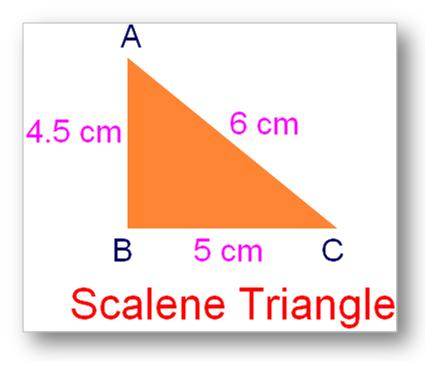AC > BC >AB

6 cm > 5 cm > 4.5 cm.

2. Isosceles Triangle:

A triangle in which two of its sides are equal is called isosceles triangle.

Isosceles Triangle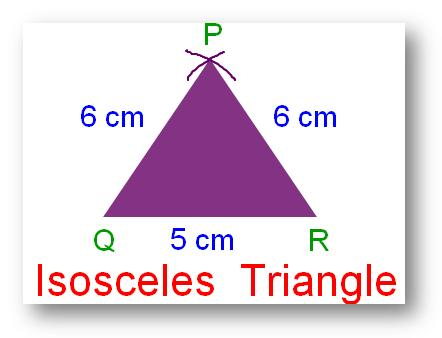PQ = PR = 6 cm.

3. Equilateral Triangle:

A triangle in which have all its three sides equal in length is called an equilateral triangle.

Equilateral Triangle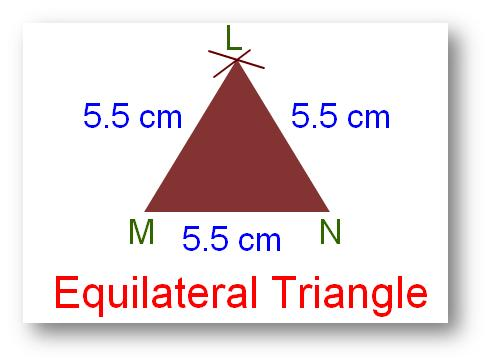LM = MN = NL = 5.5 cm

. Classify the triangle into acute triangle, obtuse triangle and right triangle with the following angles:

(a) 90°, 45°, 45°

(b) 60°, 60°, 60°

(c) 80°, 60°, 40°

(d) 130°, 40°, 10°

(e) 90°, 35°, 55°

(f) 92°, 38°, 50°

3. Classify the triangle according to sides, that is, equilateral, isosceles and scalene triangles

(a) 6 cm, 3 cm, 5cm.

(b) 6 cm, 6 cm, 6 cm.

(c) 7 cm, 7 cm, 5 cm.

(d) 8 cm, 12 cm, 10 cm.

(e) 3 cm, 4 cm, 5 cm.

(f) 3.5 cm, 3.5 cm, 4.5 cm.

4. Is it possible to have a triangle with the following angles and sides? Give reason in support of your answer.

(a) 110°, 60°, 30°

(b) 70°, 70°, 70°

(c) 80°, 35°, 65°

(d) 7 cm, 3 cm, 4 cm.

(e) 50°, 50°, 90°

(f) 10 cm, 12 cm, 2 cm.

5. Find the perimeter of a triangle when its sides are:

(a) AB = 7.6 cm, BC = 4.5 cm, CA = 6.3 cm.

(b) PQ = 4.00, QR = 3 cm, RP = 5 cm.

(c) LM = 4.5 cm, MN = 3.6 cm, NL = 6.2 cm.

6. If in a triangle LMN:

(a) ∠L = 80°, ∠M = 50°, find ∠N,

(b) ∠M = 60°, ∠N = 60°, find ∠L,

(c) ∠M = 100°, ∠ N = 30°, find ∠ L,

(d) ∠N = 90°, ∠L = 45°, find ∠M.

Mention the kind of triangle also.

7. The angle of a triangle is in the ratio of 2: 3: 4. Find the measure of each angle of the triangle.

8. One of the two equal angles of an isosceles triangle measures 55°. Determine the other angles.

9. The perimeter of a triangle is 24 cm. Two of its sides are 8 cm and 9 cm. Find the length of its third side.

10. Each side of a ∆ is one third of its perimeter. What kind of triangle is this?

11. One of the acute angles of a right triangle is 48°. Find the other acute angle.

12. Say whether the following statements are true or false:

(a) All the angles of an isosceles triangle are equal.

(b) If one angle of a triangle is obtuse, the other two angles must be acute.

(c) A right triangle can be equilateral.

(d) Equiangular triangle has its three sides also equal.

(e) A triangle can have two obtuse angles.

Drawing polygons not exceeding octagons

1. Acute Angle:

An angle whose measure is less than 90° is called an acute angle.

Acute Angle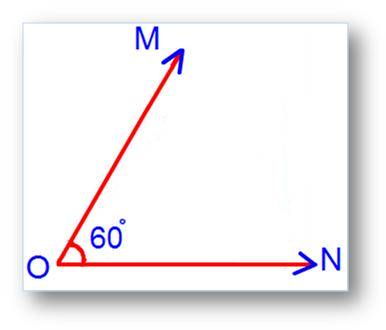∠MON shown in adjoining figure is equal to 60°. So, ∠MON is an acute angle.

2. Right Angle:

An angle whose measure is 90° is called right angle.

Right Angle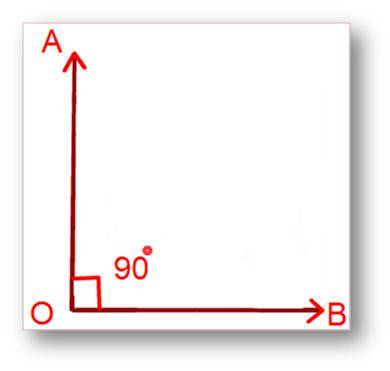In the above figure, ∠AOB is a right angle. In this case, we say that the arms OA and OB are perpendicular to each.

Therefore, ∠AOB shown in adjoining figure is 90°.

So, ∠AOB is a right angle.

3. Obtuse Angle: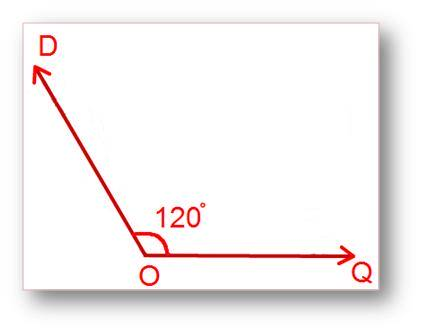An angle whose measure is greater than 90° but less than 180° is called an obtuse angle.

Obtuse Angle

6Save

∠DOQ shown in the above figure is an obtuse angle.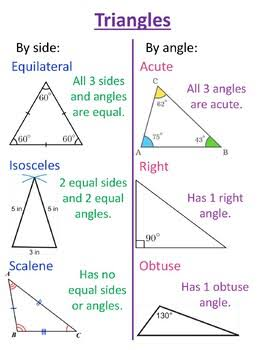Quadrilaterals

These are 4-sided plane shapes. They are rectangles, parallelograms, squares, rhombus,

kite and trapezium.

Rectangles

Opposite sides are equal.

Each of the interior angle is 90°.

Opposite sides are parallel

AB // CD

AD // BC

The two diagonals are AC and BD. Is AC BD?

The two lines of symmetry are MN and ST. Is MN ST?

Parallelograms

Opposite sides are equal and parallel.

A B

D C

None of the angles is equal to 90°

Is diagonal AC BD?

How many lines of symmetry has parallelogram ABCD?

Squares

Opposite sides are equal parallel.

A B

D C

AB CD AD BC

All the sides are equal.

Each of the interior angle is 90°.

Draw the lines of symmetry. How many are they?

Is AC BD?

Rhombus

Opposite sides are equal and parallel. A B

C D

AB CD and AD BC

All the sides are equal.

AB CD AD BC

None of the angles is equal to 90°.

Draw the diagonals AC and BD.

Measure AC and BD.

Is AC BD?

How many lines of symmetry does a rhombus have?

Kite

Opposite sides are not equal.

How many lines of symmetry does a kite have?

Name them.

Draw the diagonals. How many are they?

Name the diagonals.

None of the interior angles is equal to 90°.

.

Trapezium

A pair of the opposite sides are parallel.

Two of the interior angles may be 90°.

How many lines of symmetry does a trapezium have?

These are two different types; see fig 1 and fig 2.

Draw the diagonals. How many are they?

Pentagon

A regular pentagon has 5 equal sides

and angles.

Here AB BC CD DE EA.

How many diagonals does a pentagon

have?

How many lines of symmetry does a

pentagon have?

Hexagon

A regular hexagon has 6 equal sides and

angles.

How many lines of symmetry does pentagon ABCDEF have?

Heptagon

A regular heptagon has 7 equal sides and angles.

Octagon

A regular octagon has 8 equal sides and

angles.

.

Properties of different prisms

Properties of a cube

It has 6 equal faces. Each face is a square

It has 12 straight edges

All the edges are equal

It has 8 vertices (corners).

Properties of a cuboid

A cuboid has 6 faces

Some faces are either a

square or a rectangle

It has 12 straight edges

It has 8 vertices (corners).

Properties of a cylinder

A cylinder has 2 plane faces

The 2 plane faces are circular

It has 1 curved face

It has 2 curved edges.

Properties of a triangular prism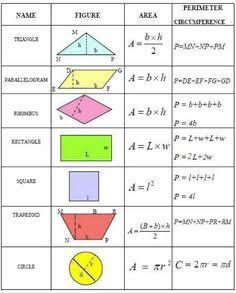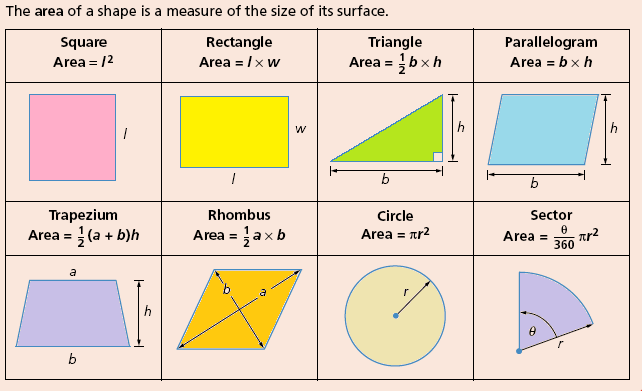Presentation

The topic is presented step by step

Step 1:

The class teacher revises the previous topics

Step 2.

He introduces the new topic

Step 3:

The class teacher allows the pupils to give their own examples and he corrects them when the needs arise

Evaluation :

Assignment :

Prepare for the next lesson by reading about

POLYGON AND PLANE FIGURES

(Visited 256 times, 6 visits today)
error: Content is protected !!